# 2nd PUC Maths Question Bank Chapter 10 Vector Algebra Ex 10.2

Students can Download Maths Chapter 10 Vector Algebra Ex 10.1 Questions and Answers, Notes Pdf, 2nd PUC Maths Question Bank with Answers helps you to revise the complete Karnataka State Board Syllabus and score more marks in your examinations.

## Karnataka 2nd PUC Maths Question Bank Chapter 10 Vector Algebra Ex 10.2

### 2nd PUC Maths Vector Algebra NCERT Text Book Questions and Answers Ex 10.2

Question 1.
Compute the magnitude of the following vectors:

$$\overrightarrow{\mathbf{a}}=\hat{\mathbf{i}}+\hat{\mathbf{j}}+\hat{\mathbf{k}}$$
Answer: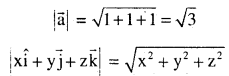$$\overrightarrow{\mathbf{b}}=2 \hat{\mathbf{i}}-7 \hat{\mathbf{j}}-3 \hat{\mathbf{k}}$$
Answer: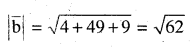$$\overrightarrow{\mathrm{c}}=\frac{1}{\sqrt{3}} \hat{\mathbf{i}}+\frac{1}{\sqrt{3}} \hat{\mathbf{j}}-\frac{1}{\sqrt{3}} \hat{\mathbf{k}}$$
Answer: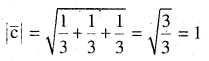Question 2.
Write two different vectors having same magnitude.
Answer: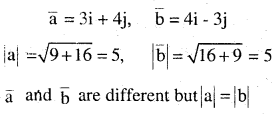Question 3.
Write two different vectors having same direction.
Answer: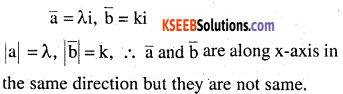Question 4.
Find the values of x and y so that the vectors $$2 \hat{\mathbf{i}}+3 \hat{\mathbf{j}} \text { and } \mathbf{x} \hat{\mathbf{i}}+\mathbf{y} \hat{\mathbf{j}}$$ are equal
Answer:
2i + 3j = xi + yj ⇒ x = 2, and y = 3

Question 5.
Find the scalar and vector components of the vector with initial point (2, 1) and terminal point (-5,7).
Ans:
Required vectors whose initial point (x1 y1)and terminal point
(x2, y2) is (x2 – x1) i + (y2-y1)j
= – 7i + 6j
Scalar components are -7 and 6 and
Vector components are -7i, 6jQuestion 6.
Find the sum of the vector
$$\overrightarrow{\mathbf{a}}=\hat{\mathbf{i}}-2 \hat{\mathbf{j}}+\hat{\mathbf{k}}$$ $$\overrightarrow{\mathbf{b}}=-2 \hat{\mathbf{i}}+4 \hat{\mathbf{j}}+5 \hat{\mathbf{k}} \text { and } \overrightarrow{\mathbf{c}}=\hat{\mathbf{i}}-6 \hat{\mathbf{j}}-7 \hat{\mathbf{k}}$$
Answer: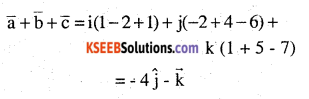Question 7.
Find the unit vector in the direction of the vector
$$\overrightarrow{\mathbf{a}}=\hat{\mathbf{i}}+\hat{\mathbf{j}}+2 \hat{\mathbf{k}}$$
Answer: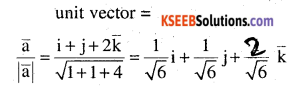Question 8.
Find the unit vector in the direction of vector  where P and Q are the points (1, 2, 3) and (4, 5, 6), respectively.
Answer: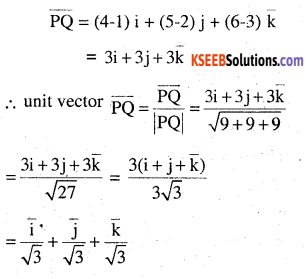Question 9.
For given vector
$$\overrightarrow{\mathrm{a}}=2 \hat{\mathrm{i}}-\hat{\mathrm{j}}+2 \hat{\mathrm{k}} \text { and } \overrightarrow{\mathrm{b}}=-\hat{\mathrm{i}}+\hat{\mathrm{j}}-\hat{\mathrm{k}}$$ and the unit vector in the direction of the vector $$\vec{a}+\vec{b}$$
Answer: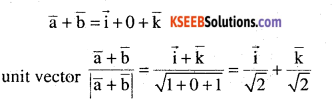Question 10.
Find a vector in the direction of vector $$5 \hat{\mathbf{i}}-\hat{\mathbf{j}}+2 \hat{\mathbf{k}}$$ which has magnitude 8 units
Answer: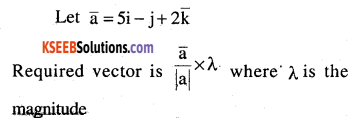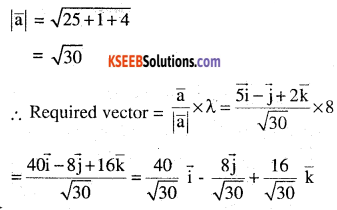Question 11.
Show that the vector
$$2 \hat{\mathbf{i}}-3 \hat{\mathbf{j}}+4 \hat{\mathbf{k}} \text { and }-4 \hat{\mathbf{i}}+6 \hat{\mathbf{j}}-8 \hat{\mathbf{k}}$$ are collinear.
Answer: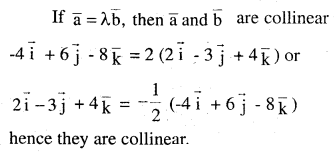Question 12.
Find the direction cosines of the vector $$\hat{\mathbf{i}}+2 \hat{\mathbf{j}}+3 \hat{\mathbf{k}}$$
Answer: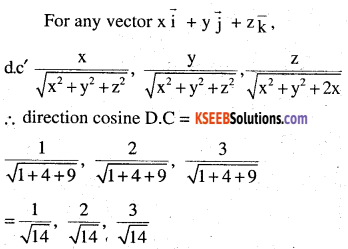Question 13.
Find the direction cosines of the vector joining the points A (1, 2, -3) and B (-1, -2, 1), directed from A to B.
Answer: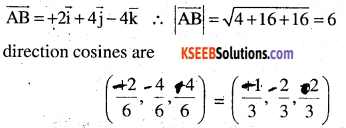Question 14.
Show that the vector $$\hat{\mathbf{i}}+\hat{\mathbf{j}}+\hat{\mathbf{k}}$$ is equally inclined to the axes OX,OY and OZ
Answer: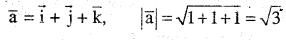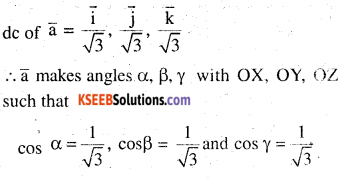Question 15.
Find the position vector of a point R which divides the line joining two points P and Q
whose position vectors are $$\hat{\mathbf{i}}+2 \hat{\mathbf{j}}-\hat{\mathbf{k}} \text { and }-\hat{\mathbf{i}}+\hat{\mathbf{j}}+\hat{\mathbf{k}}$$ respectively, in the ratio 2:1
(i) Internally
(ii) Externally
Answer: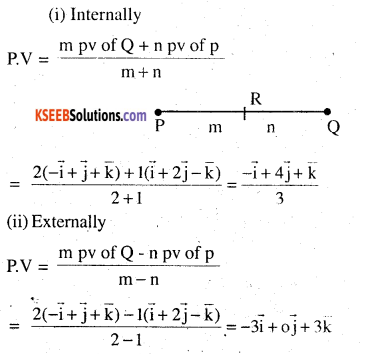Question 16.
Find the position vector of the mid point of the vector joining the points P (2, 3, 4) and Q (4, 1, -2).
Answer: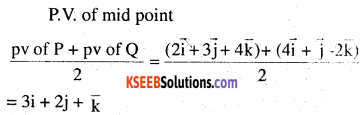Question 17.
Show that the points A, B and C with position vectors,
$$\overrightarrow{\mathrm{a}}=3 \hat{\mathrm{i}}-4 \hat{\mathrm{j}}-4 ,\overrightarrow{\mathbf{b}}=2 \hat{\mathbf{i}}-\hat{\mathbf{j}}+\hat{\mathbf{k}} \text { and } \quad \overrightarrow{\mathbf{c}}=\hat{\mathbf{i}}-3 \hat{\mathbf{j}}-5 \hat{\mathbf{k}}$$
Answer: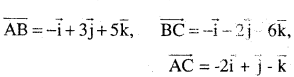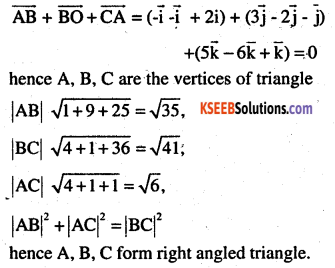Question 18.
In triangle ABC (Fig 10.18), which of the following is not true: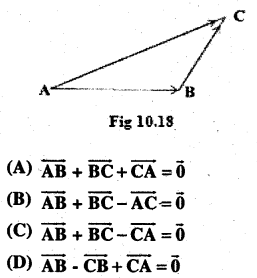Answer: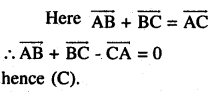Question 19.
If $$\overrightarrow{\mathbf{a}} \text { and } \overrightarrow{\mathbf{b}}$$
are two collinear vectors,then which of the following are incorrect:
(A) $$\overrightarrow{\mathbf{b}}=\lambda \overrightarrow{\mathbf{a}}$$ for some scalar λ
(B) $$\overrightarrow{\mathbf{a}}=\pm \overrightarrow{\mathbf{b}}$$
(C) the respective components of $$\overrightarrow{\mathbf{a}} \text { and } \overrightarrow{\mathbf{b}}$$ are properties.
(D) both of the vectors $$\overrightarrow{\mathbf{a}} \text { and } \overrightarrow{\mathbf{b}}$$ have same direction ,but different magnitudes
Answer:
By definition of collinear vectors
(A), (B) and (C) are true.
Hence Answer (D).